# Inter solution forMaths 1A exercise 6(b) first year

Intermediate first year mathematics solutions for trigonometric ratios upto transformations exercise 6(b) are given.

The solutions are very easy to understand.First you study text book lesson very well.

Observe the example problems and solutions. Try them. Observe the given solutions and try them in your own method.

You can also see

Inter Maths 1A textbook solutions

Inter Maths 1B textbook solutions

Inter Maths IIA textbook solutions

Inter Maths IIB textbook solutions

Trigonometric Ratios upto Transformations

Exercise 6(a)

Exercise 6((b)

Exercise 6(c)

Exercise 6(d)

Exercise 6(e)

Exercise 6(f)

M

## Trigonometric ratios upto transformations solutions for exercise 6(b) inter 1A

Exercise 6(b)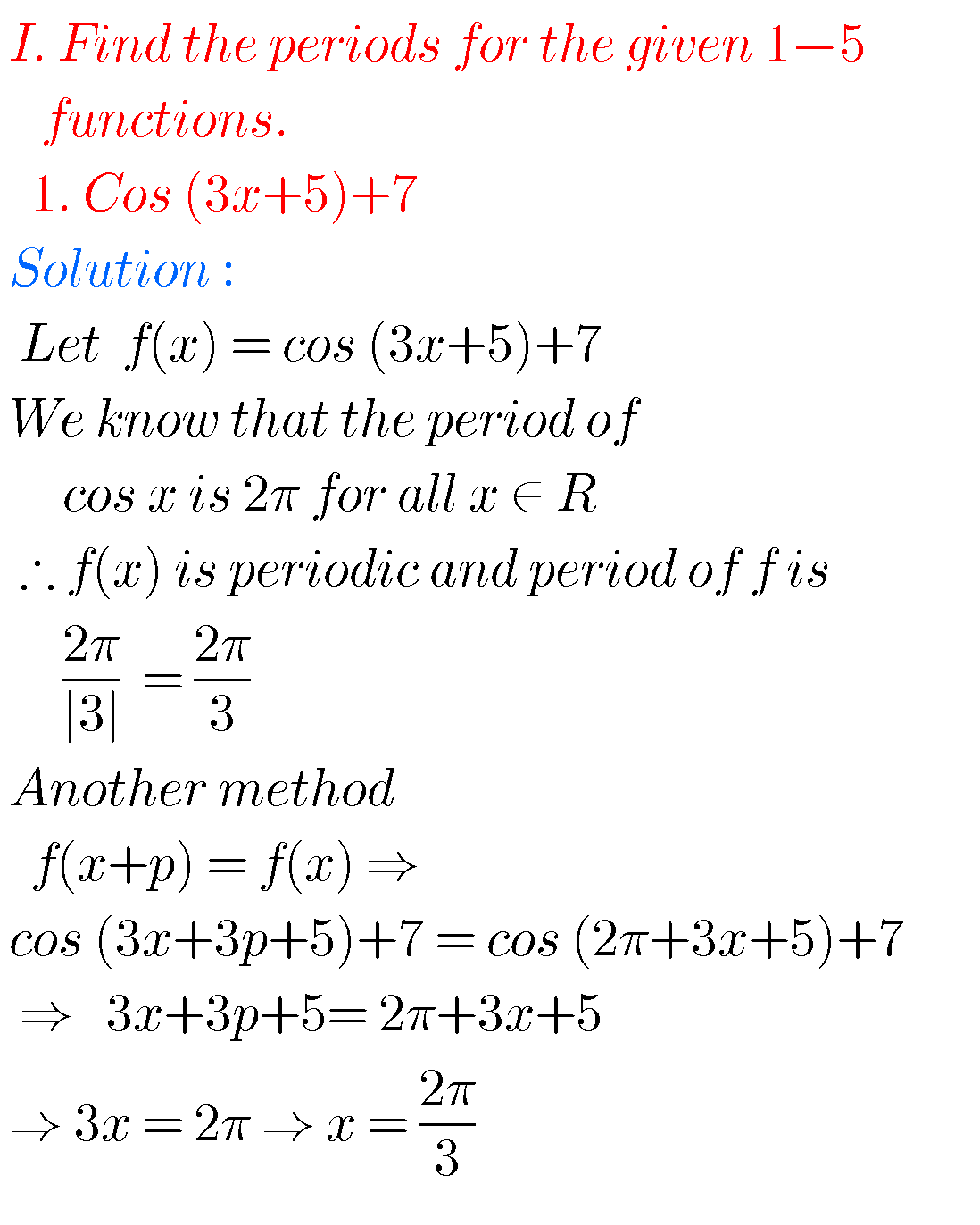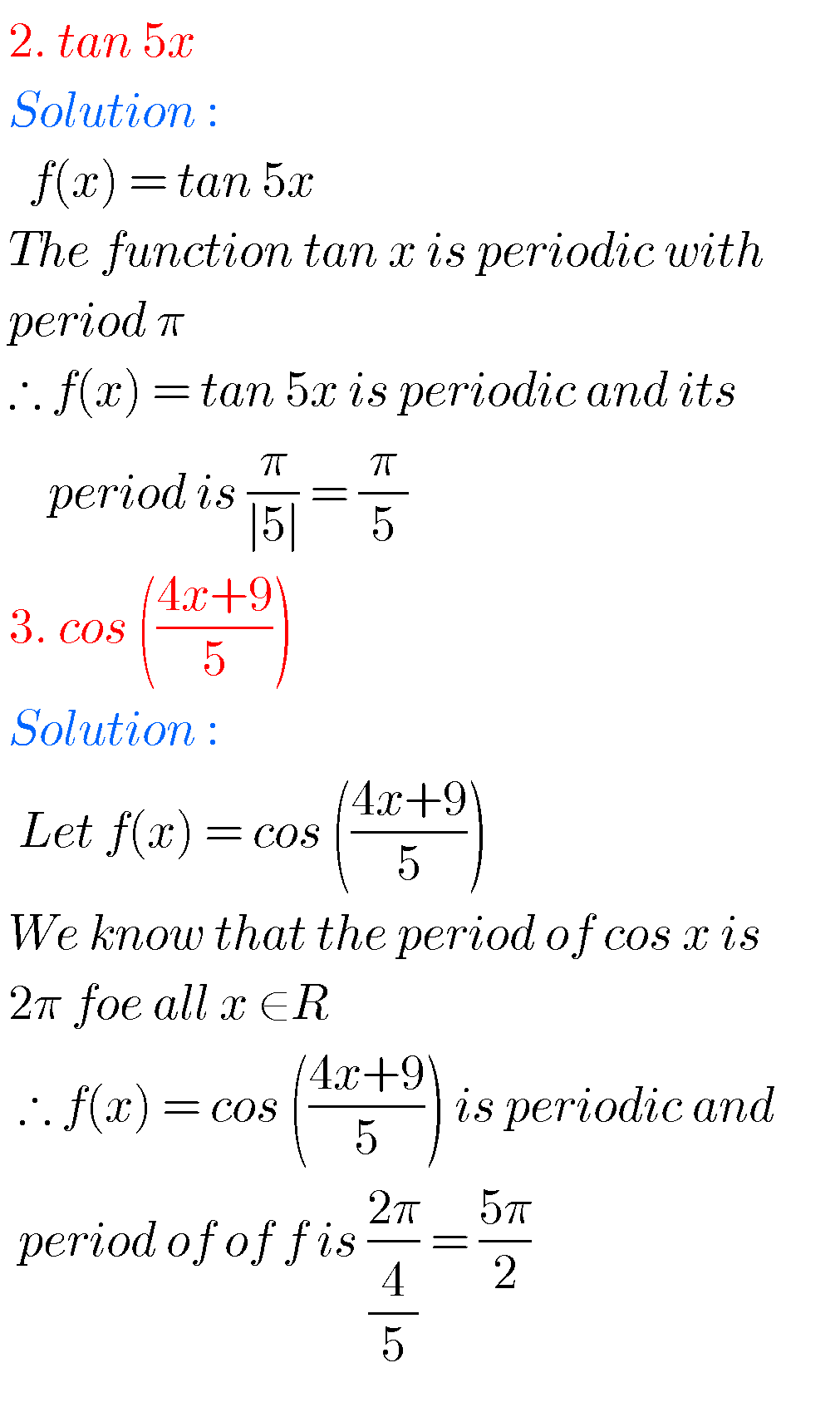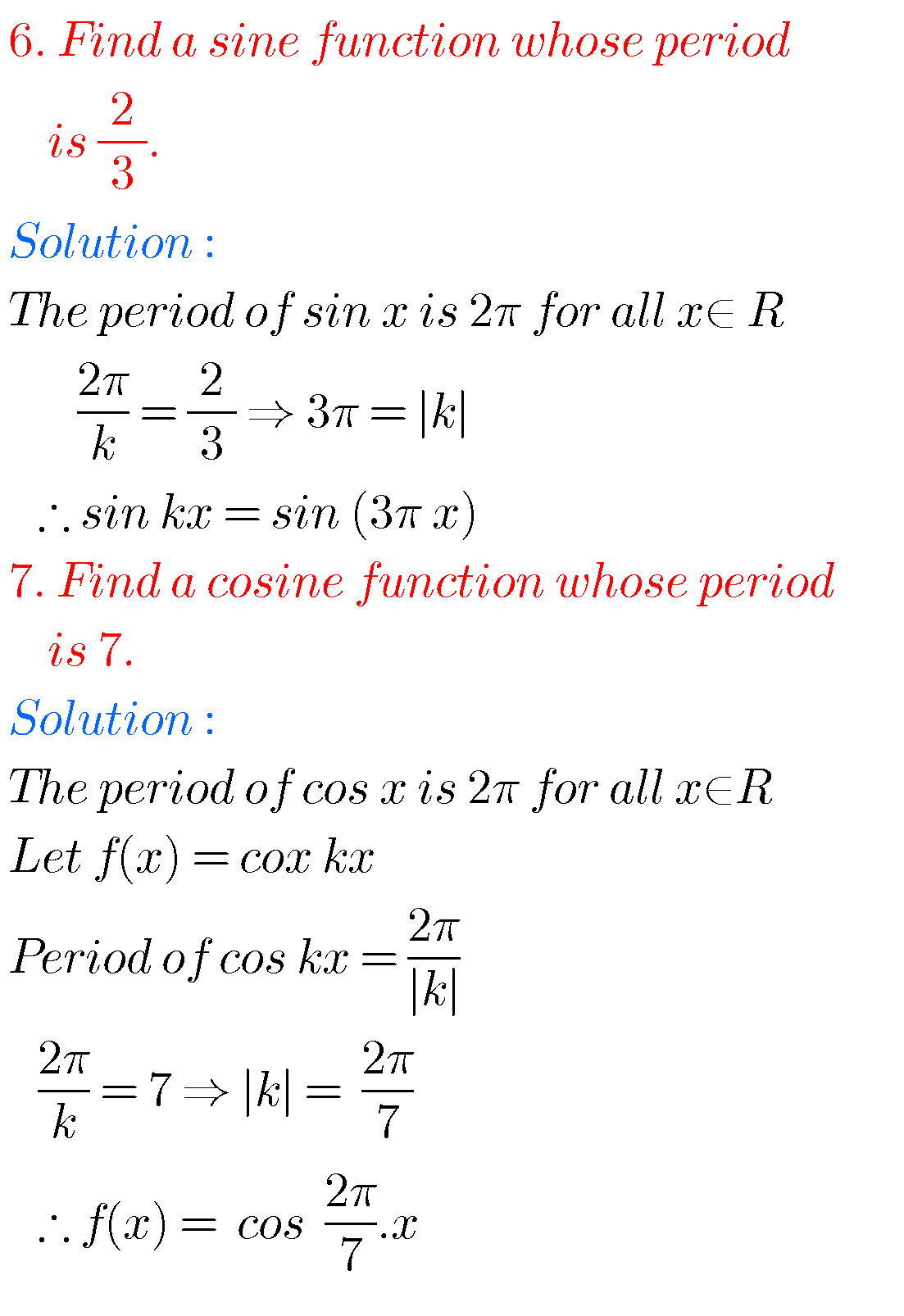Figure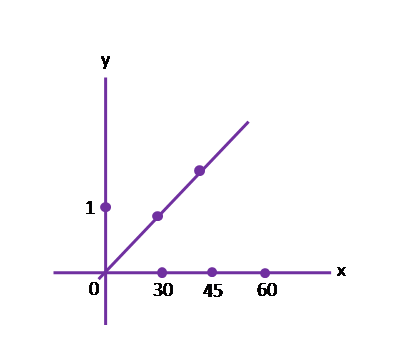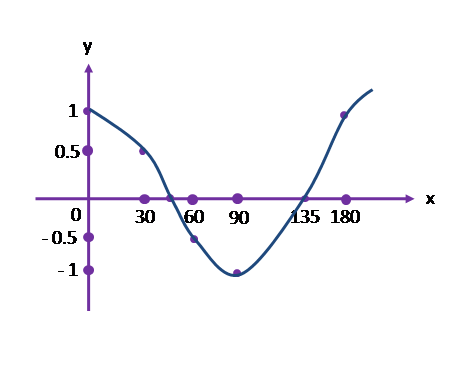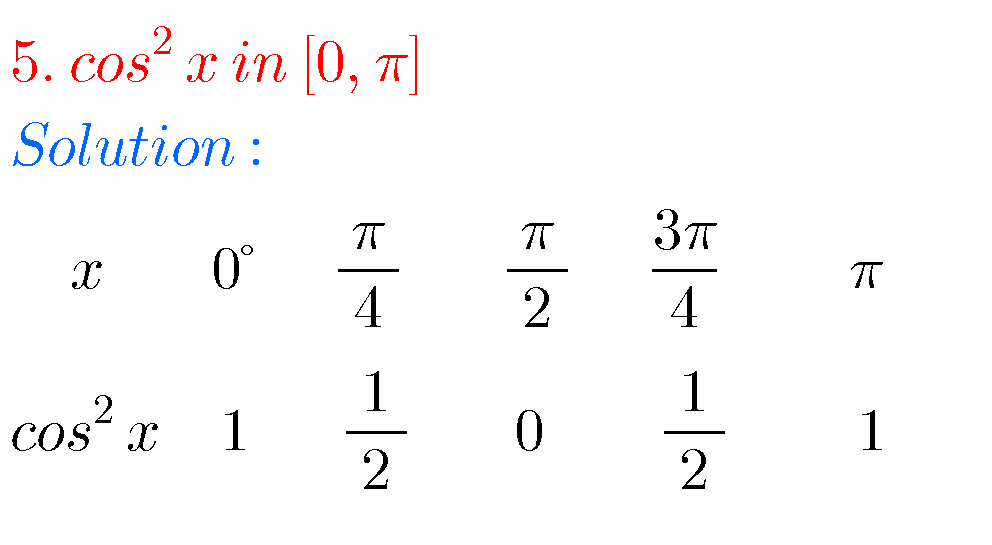Note :

Observe the solutions and try them in your own methods.

Some moreYou can see the

Inter IIA complex numbers solutions

Inter IIA De Moivre’s theorem solutions

Class 10 maths real numbers solutions

Class 10 maths sets solutions

Ncert solutions for class 8 linear equations in one variable solutions

### 2 thoughts on “Inter maths solutions for exercise 6(b) first year,Trigonometric Ratios upto Transformations Solutions for 6(b) IA”

1.Nice super

2.Very nice so much